## Sunday, August 21, 2011

### Using T-shirts To Teach Math Properties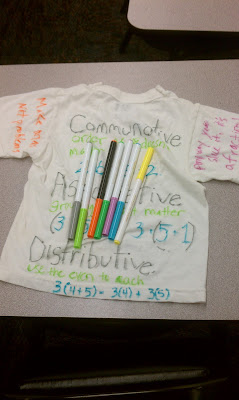(Be sure to ask your local stores for donations)

## Saturday, August 20, 2011

### Geometric Angles & Putt Putt (Math Field Trips)

Why can't we have more math fieldtirps! Kick off the new year by requesting a field trip to learn angles! And what better place to earn various angles than a putt putt corse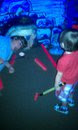(Mini Golf With My Son)

## Wednesday, August 17, 2011

### Compound and Simple Interest & Your First Home

Compound and Simple Interest & Your First Home

This is a neat activity if you need to teach compound or simple interest. Bring in one of your local real estate books (or print real estate pics online) and have your students choose which house they would like to live in. Next, have them calculate the total amount they will pay over the course of 30 years using both formulas (if you are teaching younger students who need not yet learn compound interest it may still be in the interest of the teacher to calculate the same home using the compound interest formula)

﻿

## Sunday, August 14, 2011

### Playing Squares with Addition, Multiplication, Division & Subtraction

Playing Squares with Addition, Multiplication, Division & Subtraction

Here is another great resource from Mathwise. The game is played normally, like you would play squares (for an example click here) but once the students complete a square using lines they must answer the math question inside the square to get points. If they answer incorrectly, no one get the points.

## Saturday, August 13, 2011

### Word Search Math: Another Wonderful Exercise From Mathwise

Mathwise offers some great exercises. This particular exercise involves a number grid and your choice of addition, multiplication, subtraction or division used with an equal symbol

Simply create, or print off, a number grid and give your students the rules of play

In the exercise below, the students may only use the multiplication symbol and the equal symbol

The students search for numbers that could be multiplied. Next they add the “X” and the “=” symbol and circle.

## Thursday, August 11, 2011

### Sample Space, Clothing and Outfits

Normally a discussion on probability or statistics begins with outcomes from an experiment, what we refer to as sample space

Taken From CNX.org

sample space is a set or collection of outcome of a particular random experiment.
For example, imagine a dart board. You are trying to find the probability of getting a bullseye. The dart board is the sample space. The probability of a dart hitting the dart board is 1.0. For another example, imagine rolling a six sided die. The sample space is {1, 2, 3, 4, 5, 6}.

Examples of Sample Space.
• The tossing of a coin, sample space is {Heads, Tails}
• The roll of a die, sample space is {1, 2, 3, 4, 5, 6}
• The selection of a numbered ball (1-50) in an urn, sample space is {1, 2, 3, 4, 5, ...., 50}
• Percentage of calls dropped due to errors over a particular time period, sample space is {2%, 14%, 23%, ......}
• The time difference between two messages arriving at a message centre, sample space is {0, ...., infinity}
• The time difference between two different voice calls over a particular network, sample space is {0, ...., infinity}

Many educators use a tree diagram to help students understand outcomes which is a very handy tool.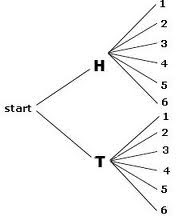(Flipping a Coin and Rolling A Die)

Yet, even with a great tool like a tree diagram, there is still a disconnect between the teaching and the student. We need a better way to reach them!

I like this idea suggested by one of my statistics students!

How Many Outfits Can I Wear?

Using stickers of different clothing (shoes, hats, dresses, etc), let your students discover the amount of mixing and matching they can perform to create different outfits.

## Wednesday, August 3, 2011

### Composite Functions and Almonds f(g(x))

Here's a tough concept for high school math students, composite functions (for more on functions click here)

• Composition of Functions is the process of combining two functions where one function is performed first and the result of which is substituted in place of each x in the other function.

Yet, what does this actually mean? And how can a young student of math make sense out of such abstract definitions. Let's call upon an analogy using almonds.

Let our ultimate goal be to have roasted almonds mixed with other assorted nuts. Thus, we have two machines; one that roasts the almonds and one that adds other assorted nuts in with the roasted almonds.

But, loosely speaking, a machine is simply a function; it transfers inputs to outputs (or in the language of math it transfers domains to ranges)(Here is a picture of our process f(g(x))(Let x, our input, be regular almonds)(We feed our regular almonds in our first machine, call it our g machine, which roasts our almonds)(Our g machine takes plain almonds and roasts them, thus, it takes almonds x and roasts them making them g(x). We then take our roasted almonds and use them as our input in the next machine)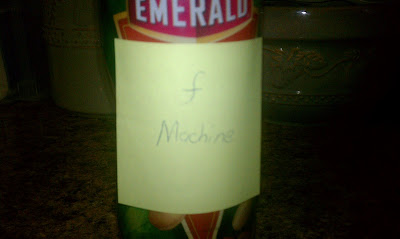(Here is out next machine, our f machine. Our f machine takes roasted almonds and adds assorted nuts )(Thus, our final output for our regular almonds after traveling through two machines is roasted almonds with assorted nuts; f(g(x))(Here is how such a concept would look in a textbook)

## Tuesday, August 2, 2011

### Number Sense For PreK-Kindergarteners

This is a cute idea created by one my Hands on Math students who teaches kindergarden.The Idea is to have your students arrange the skittles on their card to match the one given to them. After each card the students count the number of skittles they have. The goal is to arrange, for example, six skittles in a variety of different ways on a 5 x 2 card so that students become accustomed with estimating.

## Monday, August 1, 2011

### Paperclip Division With Remainders

This is a clever idea one of my students came up with for teaching division with remainders.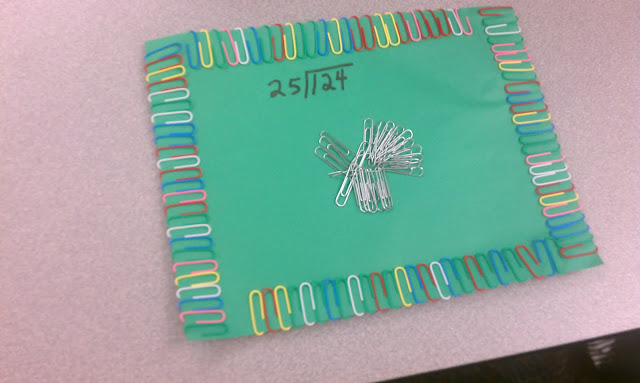The student may either place the remainder from their division in the middle page or the amount of times the number divides (not shown here). The student may then either place the remainder or the amount of times the number divides on the edges of the construction paper. This particular student decided to do clusters of paper clips around the edges in 25's.